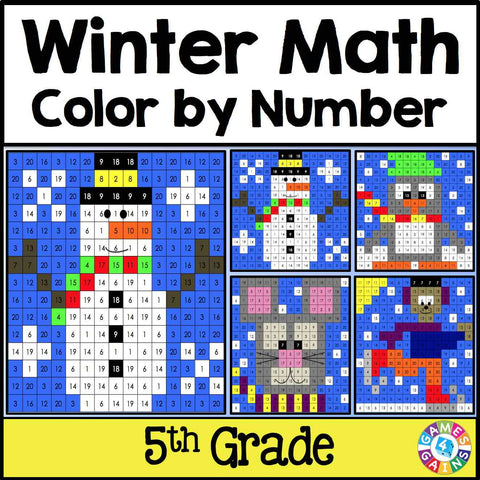Winter Math Color-by-Number - 5th Grade

• \$425

Winter Math Color-by-Number set comes with 4 winter-themed math color-by-number activities for practicing 5th grade math word problems. Included are 4 different winter math color by number activities, each requiring students to solve 20 challenging word problems. This winter math set is perfect to use in centers, in small groups, or with the whole class!

Included with this Winter Math Color-by-Number set:
Instructions
4 color by number worksheets
4 student problem worksheets
4 teacher answer sheets

Here are the skills covered by these winter activities:
• Reading and writing decimals word problems (one-step)
• Rounding and comparing decimals word problems (one-step)
• Adding and subtracting decimals word problems (one-step)
• Multiplying and dividing decimals word problems (one-step)

**PLEASE NOTE: These color-by-number activities are slightly different from my other versions. Instead of simply coloring the answer, students have to look at the key to determine which color to color the problem number in the picture. This often takes a bit more concentration for students to do. As the teacher, you will need to model this for your students.**

Want to learn more? Check out the preview for actual pages.